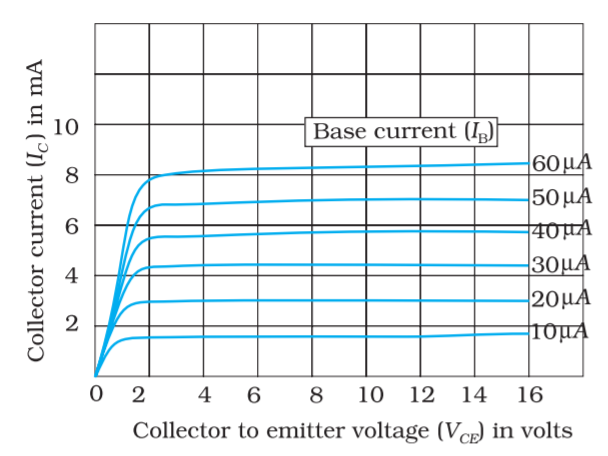Home/Class 12/Physics/

## QuestionPhysicsClass 12

From the output characteristics shown in figure. Calculate the values of $$\beta _{\mathit{ac}}$$  and $$\beta _{\mathit{dc}}$$  of the transistor when $$V_{\mathit{CE}}$$  is $$10\;V$$  and $$I_C=4.0\;\mathit{mA}$$$$\beta _{\mathit{ac}}=\left(\frac{\Delta I_C}{\Delta I_B}\right)_{V_{\mathit{CE}}},\beta _{\mathit{dc}}=\frac{I_C}{I_B}$$
For determining $$\beta _{\mathit{ac}}$$ and $$\beta _{\mathit{dc}}$$ at the stated values of $$V_{\mathit{CE}}$$  and $$I_C$$  one can proceed as follows. Consider any two characteristics for two values
of $$I_B$$  which lie above and below the given value of $$I_C.$$  Here $$I_C=4.0\mathit{mA}$$   $$($$ Choose characteristics for $$I_B=30$$  and $$20\mu \; A.$$ At $$V_{\mathit{CE}}=10$$ we read the two values of $$I_C$$  from the graph. Then
$$\Delta I_B=\left(30-20\right)\mu A=10\mu A,\Delta I_C=\left(4.5-3.0\right)\mathit{mA}=1.5\mathit{mA}$$
Therefore, $$\beta _{\mathit{ac}}=\dfrac{1.5\mathit{mA}}{10\mu A}=150$$
For determining $$\beta _{\mathit{dc}}$$  either estimate the value of $$I_B$$  corresponding to $$I_C=4.0\mathit{mA}$$  at $$V_{\mathit{CE}}=10V$$  or calculate the two values of $$\beta _{\mathit{dc}}$$  for the two characteristics chosen and find their mean. Therefore, for $$I_C=4.5\mathit{mA}$$  and $$I_B=30\mu A$$   $$\beta _{\mathit{dc}}=\dfrac{4.5\mathit{mA}}{30\mu A}=150$$
and for $$I_C=3.0\mathit{mA}$$  and $$I_B=20\mu A$$
$$\beta _{\mathit{dc}}=\dfrac{3.0\mathit{mA}}{20\mu A}=150$$
Hence, $$\beta _{\mathit{dc}}=\dfrac{(150+150)}{2}=150$$